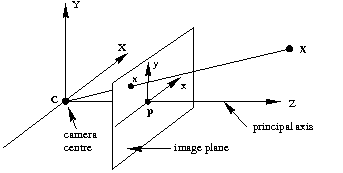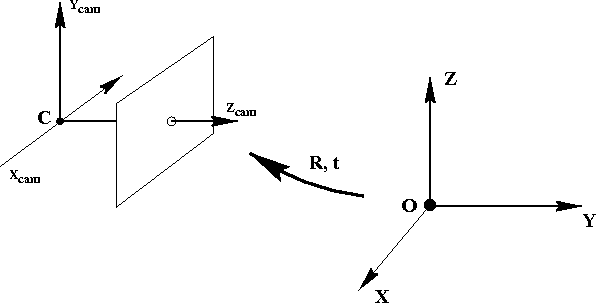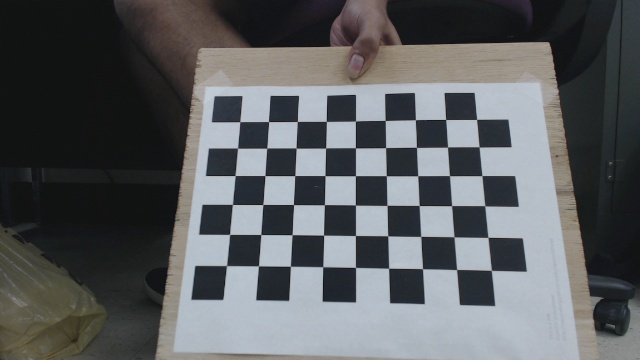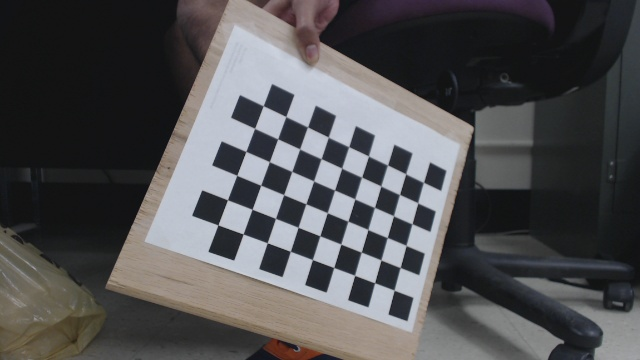# Introduction

Often for complicated tasks in computer vision it is required that a camera be calibrated. Camera calibration is a necessary step in 3D computer vision in order to extract metric information from 2D images. If you’re just looking for the code, you can find the full code here:

https://github.com/sourishg/stereo-calibration

# What is meant by calibrating a camera?

There are many lens models but for this tutorial we will assume the most commonly used pinhole model. The model is described by the two images below:The task of camera calibration is to determine the parameters of the transformation between an object in 3D space and the 2D image observed by the camera from visual information (images).

Let $\mathbf{X} = (X,Y,Z,1)^T$ be the coordinate of the point in 3D world coordinates. Then the 3D coordinate of the same point in camera frame $\mathbf{X}_{cam}$ is transformed as:

$\mathbf{X}_{cam} = \begin{bmatrix} \mathbf{R} & \mathbf{t} \end{bmatrix}\mathbf{X} \\$

where $\mathbf{R}$ is 3x3 rotation matrix and $\mathbf{t}$ is 3x1 translation matrix. Now, let $\mathbf{x}=(x,y,1)^T$ be the image coordinate of that 3D point, then the 3D to 2D mapping becomes:

$\mathbf{x} = \mathbf{K}\begin{bmatrix} \mathbf{R} & \mathbf{t} \end{bmatrix}\mathbf{X} \\$

where $\mathbf{K}$ is a 3x3 matrix containing the intrinsic parameters of the camera.

$\mathbf{K} = \begin{bmatrix} f_x & 0 & c_x \\ 0 & f_y & c_y \\ 0 & 0 & 1 \end{bmatrix}\\$

$f_x$ and $f_y$ are the focal length of the camera in the x-axis and the y-axis respectively. $(c_x, c_y)$ is coordinate of the principal point.

• Intrinsic parameters: The $\mathbf{K}$ matrix consists of all the intrinsic parameters of the camera.
• Extrinsic parameters: The $\mathbf{R}$ and $\mathbf{t}$ matrices constitutes the extrinsic parameters of the camera.

Finding out these unknown parameters is known as camera calibration. We will not delve into the complicated linear algebra involved in finding out these parameters. Here is a gist of what we’ll do to calibrate - we will take multiple images of a checkerboard with a fixed square size and find all the corner points in each image. These corner points in the image correspond to some 3D point in the world (which is easy to calculate, since the checkerboard has a very well defined geometry). We’ll store these point to point correspondences and let OpenCV use it’s non-linear algorithm to give us the calibration parameters.

# Dependencies and Datasets

You must have OpenCV 2.4.8+ and libpopt (command line args) to run the code. Also, you should have a dataset of calibration images beforehand of a fixed image resolution. Here are two sample images of the checkerboard.It is recommended to get at least 30 images of the checkerboard in all possible orientations of the checkerboard to get good calibration results.

Note: In this example, a standard 9x6 calibration board is used. The size of the square is 24.23 mm.

# Code Explained

I will only explain the important parts of the code, and you can find the full source here: https://github.com/sourishg/stereo-calibration/blob/master/calib_intrinsic.cpp

Declare all the necessary vectors to store the image points and the object points. Image points are the checkerboard corner coordinates in the image whereas object points are the actual 3D coordinate of those checkerboard points.

We create a function called setup_calibration to find all the corner points of each image and their corresponding 3D world points and prepare the object_points and image_points vectors. board_n is the total number of corner points in the checkerboard. In our example it is $9\times 6=54$. Note that we also take a bunch of args, but I hope the variable names are self explanatory.

We loop through all the images in our directory and convert them to grayscale images using the function cv::cvtColor.

Next we use the findChessboardCorners function to find all the checkerboard corners. I would suggest you go through the OpenCV documentation for more details about the arguments of this function. If the corners are found then found is set to true and the corners are further refined by the cornerSubPix function. The drawChessboardCorners function is optional, it only helps you visualize the checkerboard corners found.

Next we store the object points. Ideally we should keep the origin at the camera centre and measure the 3D points of the checkerboard corners manually but you can image how difficult it would be. So we introduce a small but beautiful hack - we keep the world origin as the top left corner point. Mathematically this doesn’t change anything (think how). Now the geometry of the checkerboard helps us find the other 3D coordinates of the corners very easily. The $Z$ coordinate is always $0$ since all the points lie on a plane. Since the square size for this example is 24.23 mm (units are important!) then the other points become $(24.23, 0, 0)$, $(48.46, 0, 0)$ and so on.

We get all the necessary user input using libpopt and call the setup_calibration function.

Now we do the actual calibration using the calibrateCamera function. K is in the matrix containing the intrinsics as described before. D contains the distortion coefficients. The distortion coefficients are used to remove any sort of distortion in the images. You can read more about the distortion coefficients here. rvecs and tvecs are the rotation and translation vectors. We also set flag to ignore higher order distortion coefficients $k_4$ and $k_5$.

It is good practice to save the camera matrix K and the distortion coefficients D in a file so that you can reuse these parameters later on without having to recalibrate. FileStorage writes the data in a YAML file.

# Building the Code

The following repository contains the full source. The file you are looking for is calib_intrinsic.cpp

https://github.com/sourishg/stereo-calibration/

I have used cmake to build the source and the README should help you build and run the program on your machine.